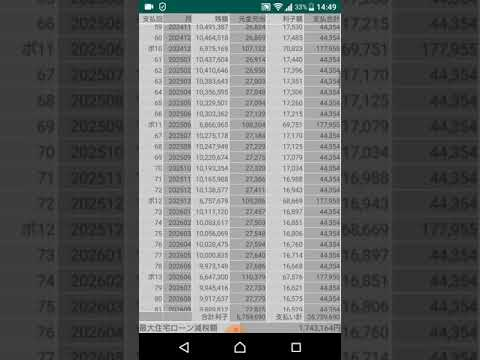# 住宅ローン計算機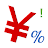500+Everyone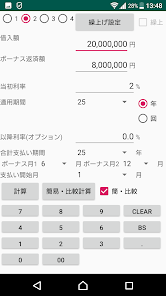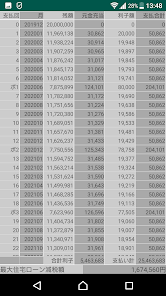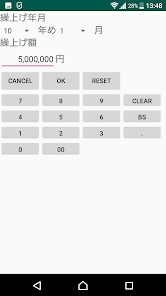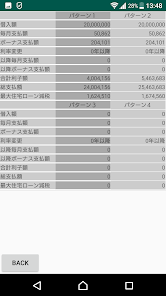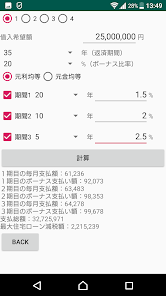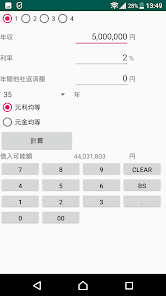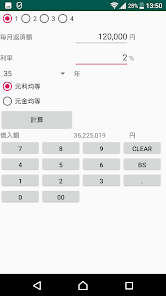This application is an application that supports the calculation of mortgages in five modes.

1 · Equal payment
It is a payment method that pays the same amount every month. Can be used in conjunction with the bonus.
Enter the principal and bonus payment amount (optional), the first interest rate, the first interest rate period, the second interest rate (optional), the total payment period, and set the bonus month. When you press the calculation button, the payment schedule will be displayed.
By pressing the advance setting button, you can set the amount of advance repayment on which month of which year. Check the advance check box to apply the advance.
※ In the case of early repayment, the second interest rate will be null and the first payment will be the total period ※
Up to 4 settings can be saved, and the Simple / Comparison check box will be displayed in the comparison list. To display the comparison list, tap the simple / compare button.
You can compare if you want to carry forward or not.

2 · Repayment of principal equally
The principal amount is divided equally by the number of payments, and the amount plus interest is the monthly payment amount.
Set the principal amount, bonus payment amount (optional), interest rate, number of payments, bonus month, and press the calculation button to display the payment schedule.
With the advance setting button, you can set how many early repayments will be made in what year of the year. Checking the advance check box enables advance payment.

Simple calculation of payment amount with interest rate of 3 ・ 3 stages
Set the principal and bonus repayment ratio, the number of years of the period, and the interest rate of each period, and select whether the principal is equal or the principal equal.
When you press the calculation button, the payment amount, bonus payment amount, total payment amount, maximum mortgage deduction amount of each institution are displayed.

4 · Calculation of the amount available for borrowing
Enter annual income and interest rate, annual amount of other people's payment, select whether it is principal equal or principal equal, and press the calculation button to display a rough estimate of the possible borrowing amount.

5 · Calculate the borrowing amount from the monthly payment amount

Enter the monthly repayment amount and interest rate, the number of years, select the principal equal or principal equal and press the calculation button to display the appropriate borrowing amount.

※ All calculation results are standard. Calculation methods differ depending on the financial institution company *
Updated on
Sep 16, 2019

## Data safety